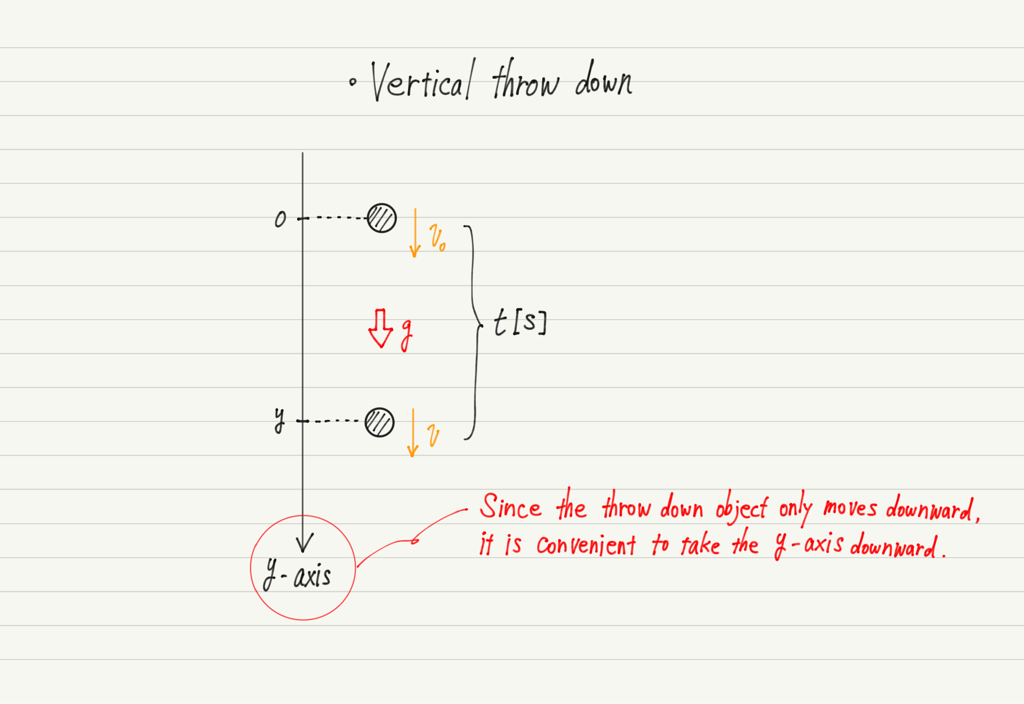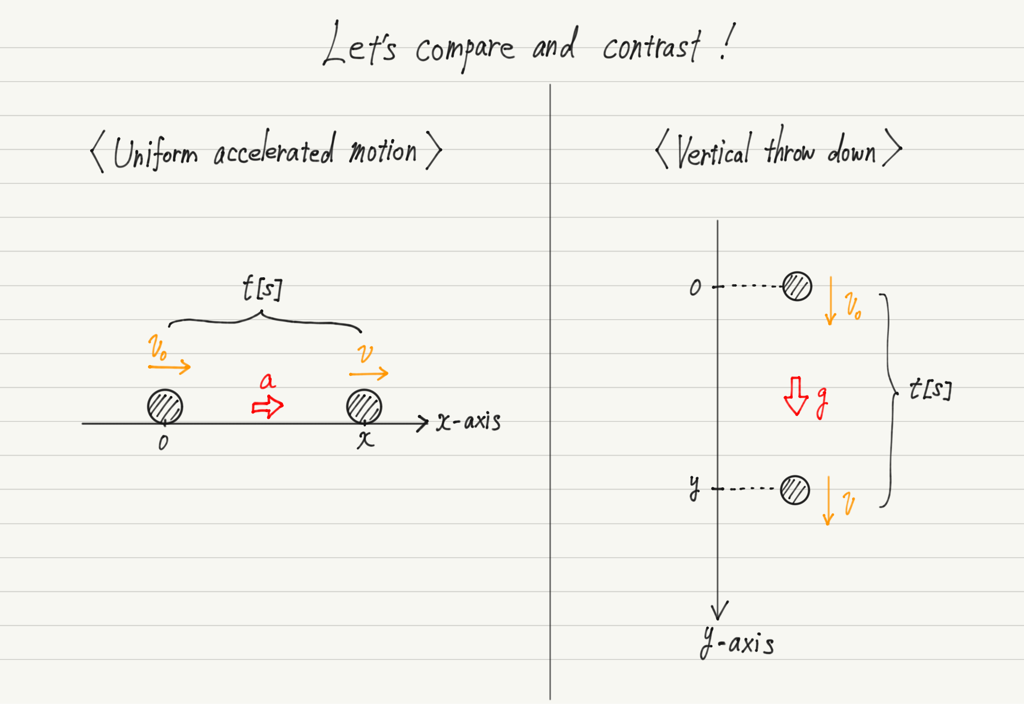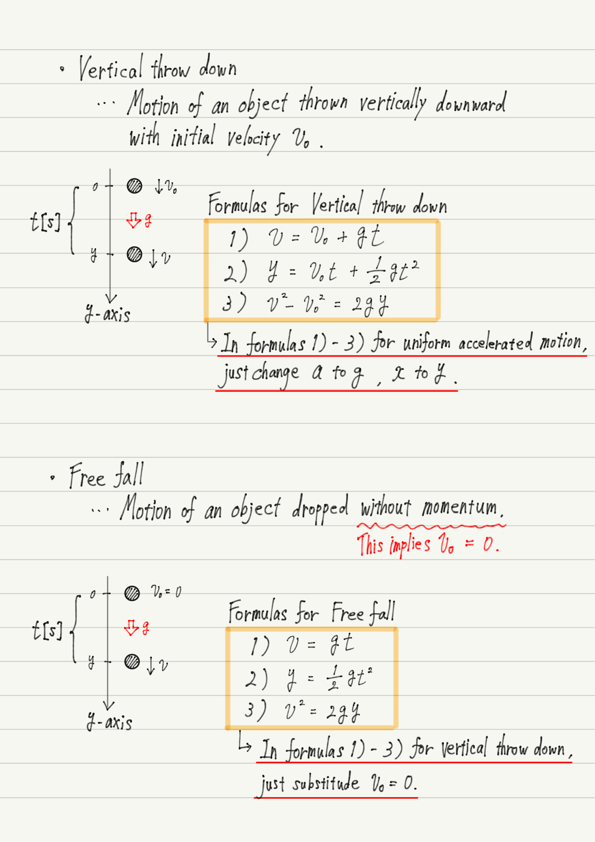# Vertical Throw Down and Free Fall

This lecture is about falling motion. As a review of the previous lecture, falling motion is a uniform accelerated motion with acceleration in g.

As long as it’s a uniform accelerated motion, the three formulas we did before should apply!

Now, let us check the specifics.

## Vertical throw down

First, consider the motion of throwing an object vertically downward.Compare this figure with the figure of uniform accelerated motion from the previous lecture.As you can see, the two figures are quite similar. Not only are they similar, they are almost identical if you change the direction of view!

The only difference is that the acceleration a is the gravitational acceleration g.
（The y-axis is used for the vertical axis; the x-axis could have been left as is, but many textbooks use the y-axis, so I followed that.）

Since the only difference is the direction, the formulas for vertical throw down and for uniform accelerated motion have the same form.

The important thing about falling motion is that “Falling motion = Uniform accelerated motion”.
In other words, all formulas for falling motion are based on the formula for uniform accelerated motion.

There are several more formulas for falling motion, but it is inefficient to memorize formulas that have the same shape each time.

Therefore, for falling motion, it is important to be able to derive the formula by yourself based on the formula for uniform acclerated motion. I have written about this in the summary note, so please check it carefully.

## Free fall

When an object is dropped quietly (with an initial velocity of 0), the motion is called “Free fall”.
In other words, a vertical throw-down motion with an initial velocity of 0 is free fall.

Since it is no different from a throw down except for the difference in initial velocity, the free-fall formula takes a much simpler form. Let’s check this in the summary note as well.

## Summary of this lecture## Next Time

Learn about the upward throwing motion in the next lecture.

https://www.yukimura-physics.com/entry/dyn-f09

error: Content is protected !!